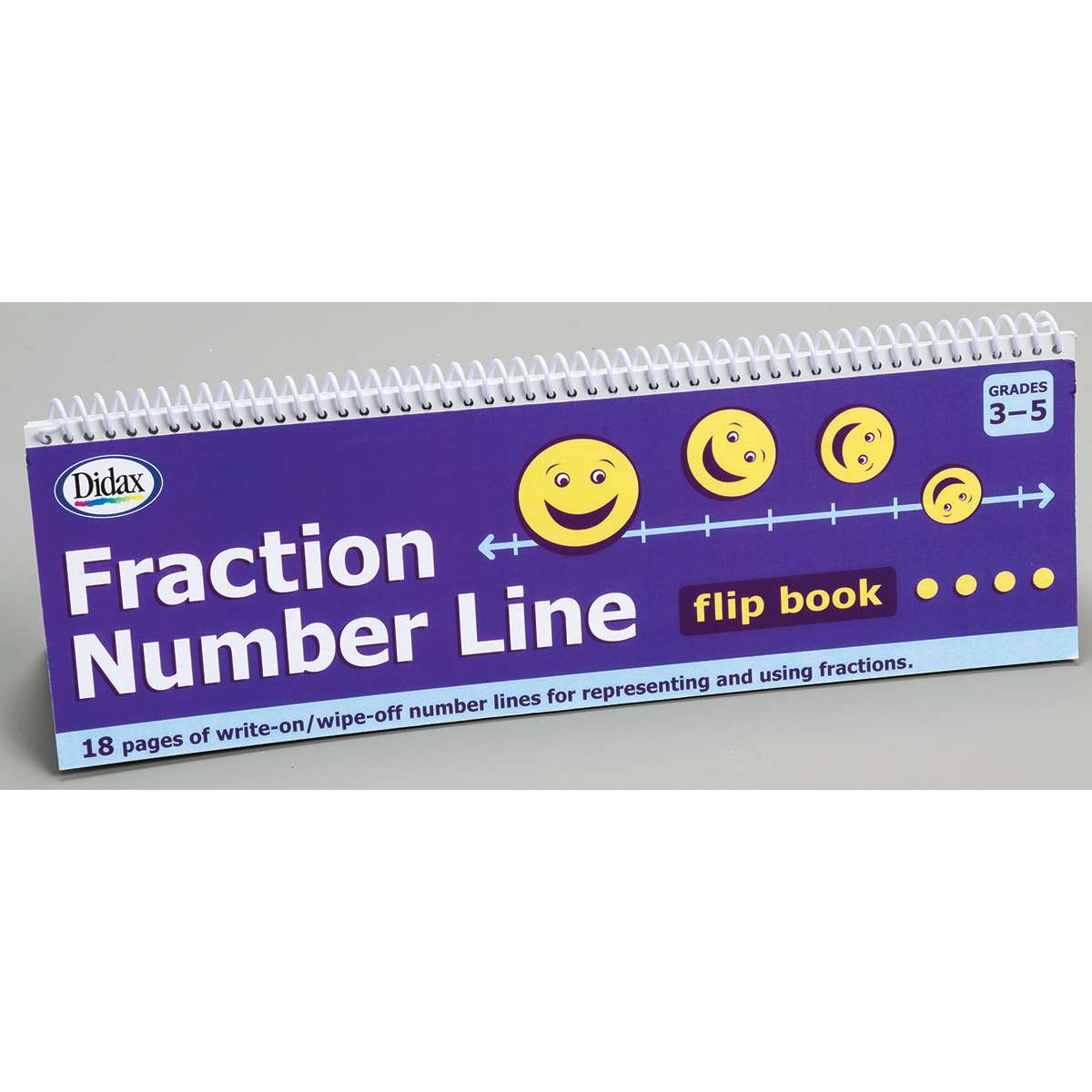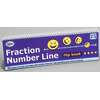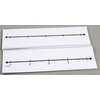••# Fraction Number Line Flip Book

Product Number: DD-211791
Students can use the 18 different fraction number lines to solve a wide variety of fraction, decimal, and percent problems. S...
Quantity

Students can use the 18 different fraction number lines to solve a wide variety of fraction, decimal, and percent problems. Spiral- bound book is 4" x 12" and includes lines featuring thirds, quarters, fifths, eighths, tenths, twelfths, sixteenths, percents, as well as blank lines (single and dual). 20 pages.
DD-211791